# 0x1. 什么是ONNX？¶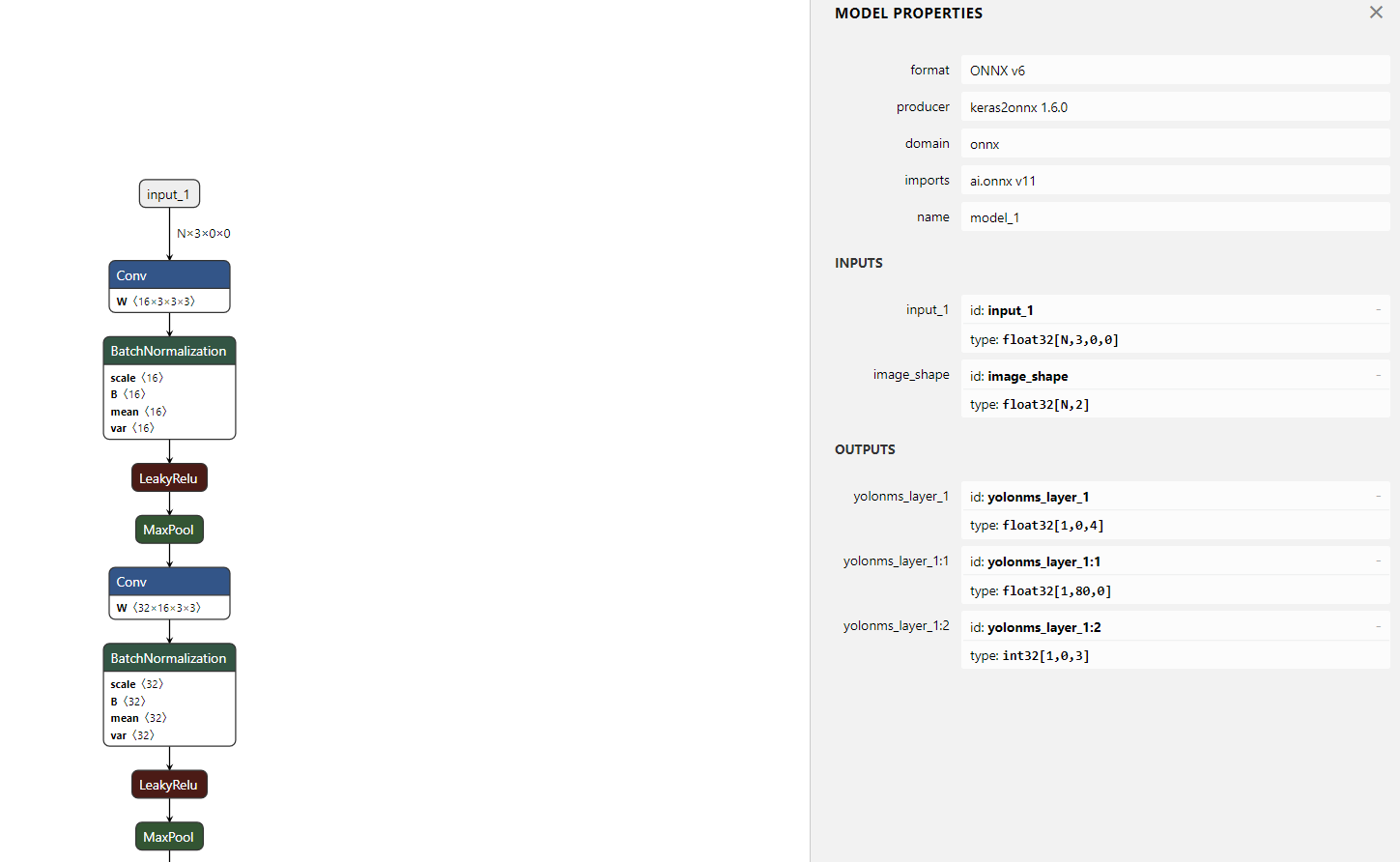# 0x2. ProtoBuf简介¶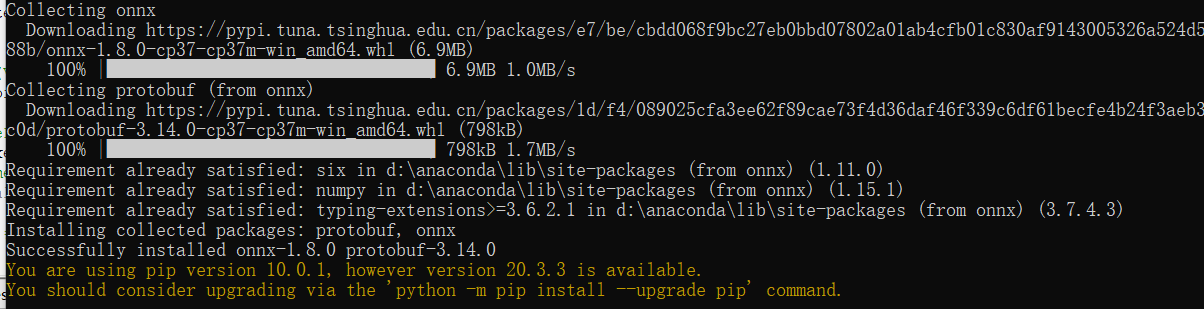Protobuf是一种轻便高效的结构化数据存储格式，可以用于结构化数据串行化，或者说序列化。它很适合做数据存储或数据交换格式。可用于通讯协议、数据存储等领域的语言无关、平台无关、可扩展的序列化结构数据格式。目前提供了 C++、Java、Python 三种语言的 API（摘自官方介绍）。

Protobuf协议是一个以*.proto后缀文件为基础的，这个文件描述了用户自定义的数据结构。如果需要了解更多细节请参考0x7节的资料3，这里只是想表达ONNX是基于Protobuf来做数据存储和传输，那么自然onnx.proto就是ONNX格式文件了，接下来我们就分析一下ONNX格式。

# 0x3. ONNX格式分析¶

• ModelProto
• GraphProto
• NodeProto
• ValueInfoProto
• TensorProto
• AttributeProto

# 0x4. onnx.helper¶

import onnx
from onnx import helper
from onnx import AttributeProto, TensorProto, GraphProto

# The protobuf definition can be found here:
# https://github.com/onnx/onnx/blob/master/onnx/onnx.proto

# Create one input (ValueInfoProto)
X = helper.make_tensor_value_info('X', TensorProto.FLOAT, [3, 2])

value = helper.make_tensor_value_info('value', AttributeProto.FLOAT, )

# Create one output (ValueInfoProto)
Y = helper.make_tensor_value_info('Y', TensorProto.FLOAT, [3, 4])

# Create a node (NodeProto) - This is based on Pad-11
node_def = helper.make_node(
['Y'], # outputs
mode='constant', # attributes
)

# Create the graph (GraphProto)
graph_def = helper.make_graph(
[node_def],
'test-model',
[Y],
)

# Create the model (ModelProto)
model_def = helper.make_model(graph_def, producer_name='onnx-example')

print('The model is:\n{}'.format(model_def))
onnx.checker.check_model(model_def)
print('The model is checked!')


# 0x5. onnx-simplifier¶

import torch

class JustReshape(torch.nn.Module):
def __init__(self):
super(JustReshape, self).__init__()

def forward(self, x):
return x.view((x.shape, x.shape, x.shape, x.shape))

net = JustReshape()
model_name = 'just_reshape.onnx'
dummy_input = torch.randn(2, 3, 4, 5)
torch.onnx.export(net, dummy_input, model_name, input_names=['input'], output_names=['output'])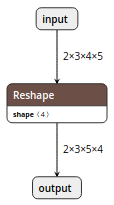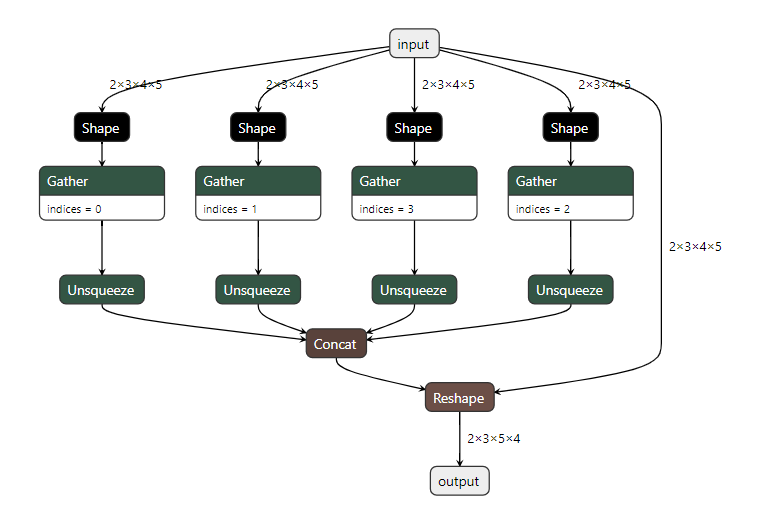def simplify(model: Union[str, onnx.ModelProto], check_n: int = 0, perform_optimization: bool = True,
skip_fuse_bn: bool = False, input_shapes: Optional[TensorShapes] = None, skipped_optimizers: Optional[Sequence[str]] = None, skip_shape_inference=False) \
-> Tuple[onnx.ModelProto, bool]:
if input_shapes is None:
input_shapes = {}
if type(model) == str:
# 加载ONNX模型
# 检查ONNX模型格式是否正确，图结构是否完整，节点是否正确等
onnx.checker.check_model(model)
# 深拷贝一份原始ONNX模型
model_ori = copy.deepcopy(model)
if not skip_shape_inference:
# 获取ONNX模型中特征图的尺寸
model = infer_shapes(model)

input_shapes = check_and_update_input_shapes(model, input_shapes)

if perform_optimization:
model = optimize(model, skip_fuse_bn, skipped_optimizers)

const_nodes = get_constant_nodes(model)
res = forward_for_node_outputs(
model, const_nodes, input_shapes=input_shapes)
const_nodes = clean_constant_nodes(const_nodes, res)
model = eliminate_const_nodes(model, const_nodes, res)
onnx.checker.check_model(model)

if not skip_shape_inference:
model = infer_shapes(model)
if perform_optimization:
model = optimize(model, skip_fuse_bn, skipped_optimizers)

check_ok = check(model_ori, model, check_n, input_shapes=input_shapes)

return model, check_ok


def infer_shapes(model: onnx.ModelProto) -> onnx.ModelProto:
try:
model = onnx.shape_inference.infer_shapes(model)
except:
pass
return model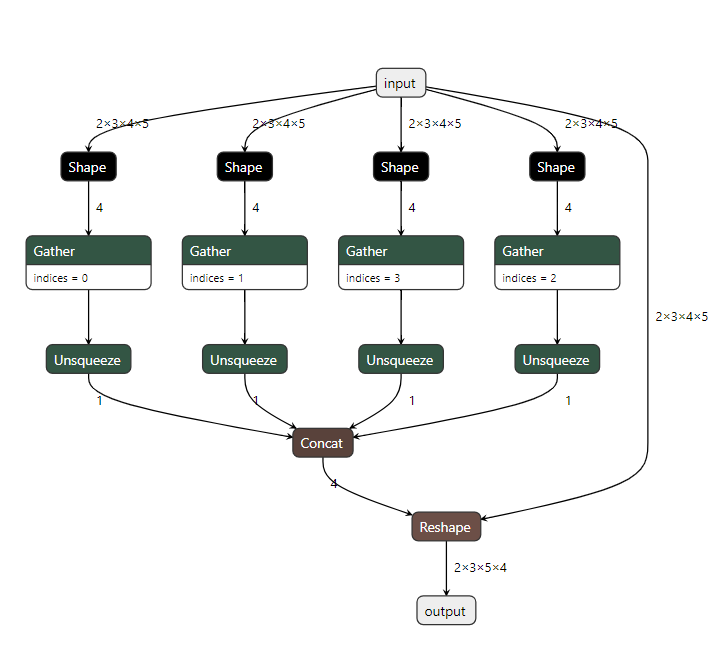def check_and_update_input_shapes(model: onnx.ModelProto, input_shapes: TensorShapes) -> TensorShapes:
input_names = get_input_names(model)
if None in input_shapes:
if len(input_names) == 1:
input_shapes[input_names] = input_shapes[None]
del input_shapes[None]
else:
raise RuntimeError(
'The model has more than 1 inputs, please use the format "input_name:dim0,dim1,...,dimN" in --input-shape')
for x in input_shapes:
if x not in input_names:
raise RuntimeError(
'The model doesn\'t have input named "{}"'.format(x))
return input_shapes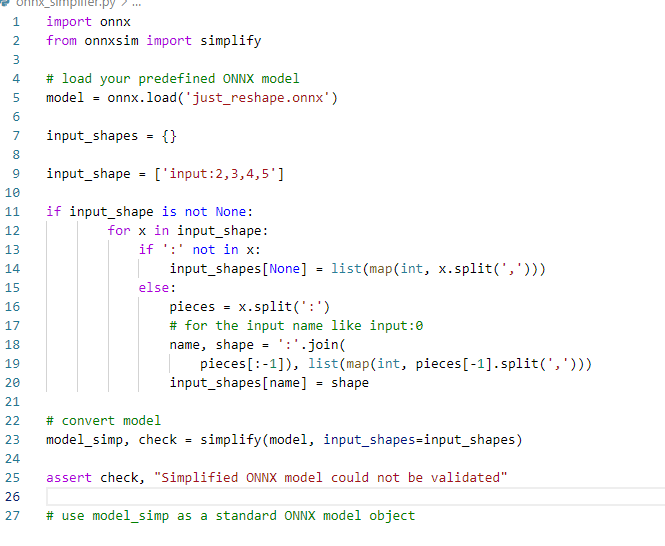def optimize(model: onnx.ModelProto, skip_fuse_bn: bool, skipped_optimizers: Optional[Sequence[str]]) -> onnx.ModelProto:
"""
:model参数: 待优化的ONXX模型.
:return: 优化之后的ONNX模型.
简化之前, 使用这个方法产生会在'forward_all'用到的ValueInfo
简化之后，使用这个方法去折叠前一步产生的常量到initializer中并且消除没被使用的常量
"""

onnx.checker.check_model(model)
onnx.helper.strip_doc_string(model)
optimizers_list = [
'eliminate_nop_dropout',
'eliminate_nop_cast',
'extract_constant_to_initializer', 'eliminate_unused_initializer',
'eliminate_nop_transpose',
'eliminate_nop_flatten', 'eliminate_identity',
'fuse_consecutive_concats',
'fuse_consecutive_log_softmax',
'fuse_consecutive_reduce_unsqueeze', 'fuse_consecutive_squeezes',
]
if not skip_fuse_bn:
optimizers_list.append('fuse_bn_into_conv')
if skipped_optimizers is not None:
for opt in skipped_optimizers:
try:
optimizers_list.remove(opt)
except ValueError:
pass

model = onnxoptimizer.optimize(model, optimizers_list,
fixed_point=True)
onnx.checker.check_model(model)
return model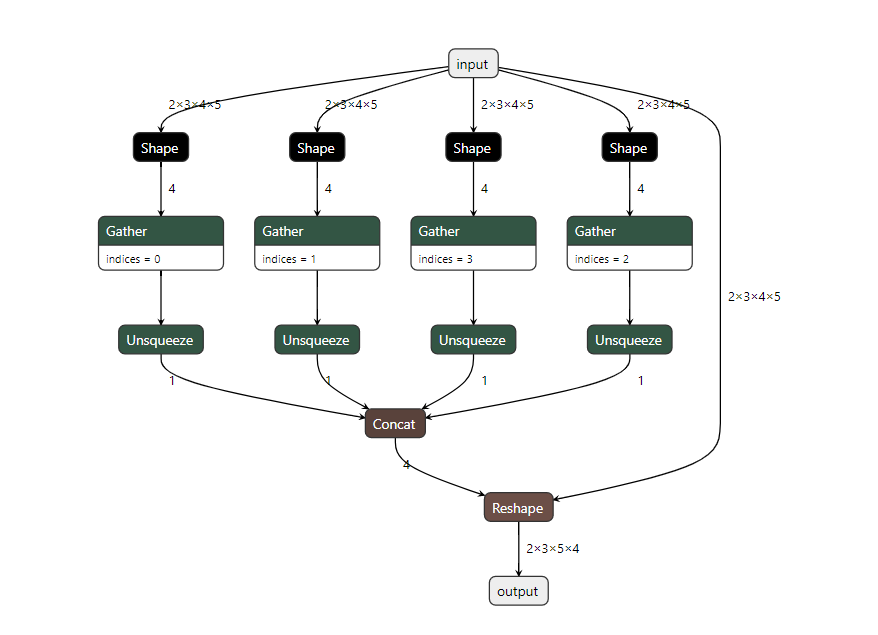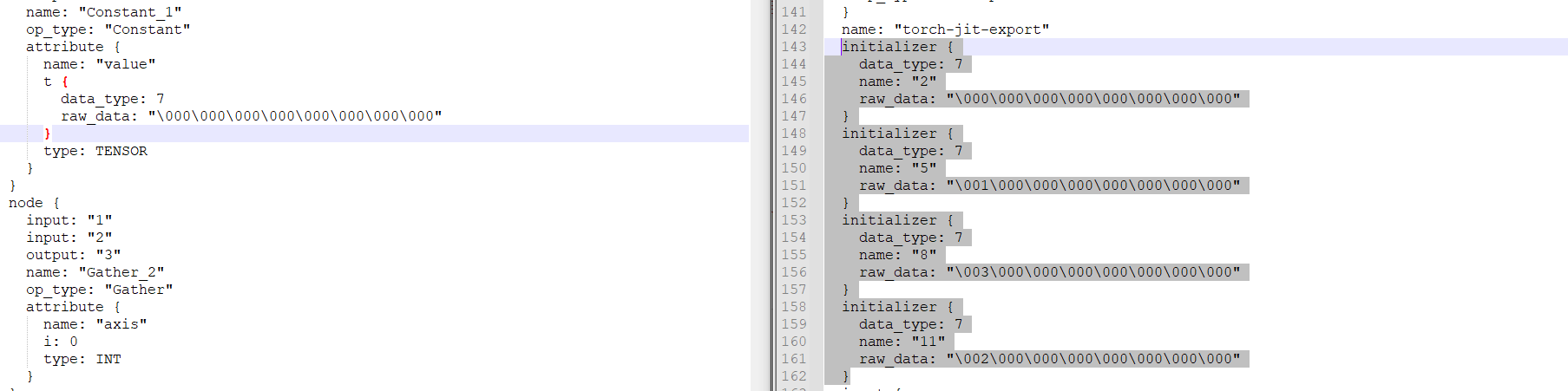def get_constant_nodes(m: onnx.ModelProto) -> List[onnx.NodeProto]:
const_nodes = []
# 如果节点的name在ONNX的GraphProto的initizlizer数组里面，它就是静态的tensor
const_tensors = [x.name for x in m.graph.initializer]
# 显示的常量OP也加进来
const_tensors.extend([node.output
for node in m.graph.node if node.op_type == 'Constant'])
# 一些节点的输出shape是由输入节点决定的，我们认为这个节点的输出shape并不是常量，
# 所以我们不需要简化这种节点
dynamic_tensors = []
# 判断是否为动态OP
def is_dynamic(node):
if node.op_type in ['NonMaxSuppression', 'NonZero', 'Unique'] and node.input not in const_tensors:
return True
if node.op_type in ['Reshape', 'Expand', 'Upsample', 'ConstantOfShape'] and len(node.input) > 1 and node.input not in const_tensors:
return True
if node.op_type in ['Resize'] and ((len(node.input) > 2 and node.input not in const_tensors) or (len(node.input) > 3 and node.input not in const_tensors)):
return True
return False
for node in m.graph.node:
if any(x in dynamic_tensors for x in node.input):
dynamic_tensors.extend(node.output)
elif node.op_type == 'Shape':
const_nodes.append(node)
const_tensors.extend(node.output)
elif is_dynamic(node):
dynamic_tensors.extend(node.output)
elif all([x in const_tensors for x in node.input]):
const_nodes.append(node)
const_tensors.extend(node.output)
# 深拷贝
return copy.deepcopy(const_nodes)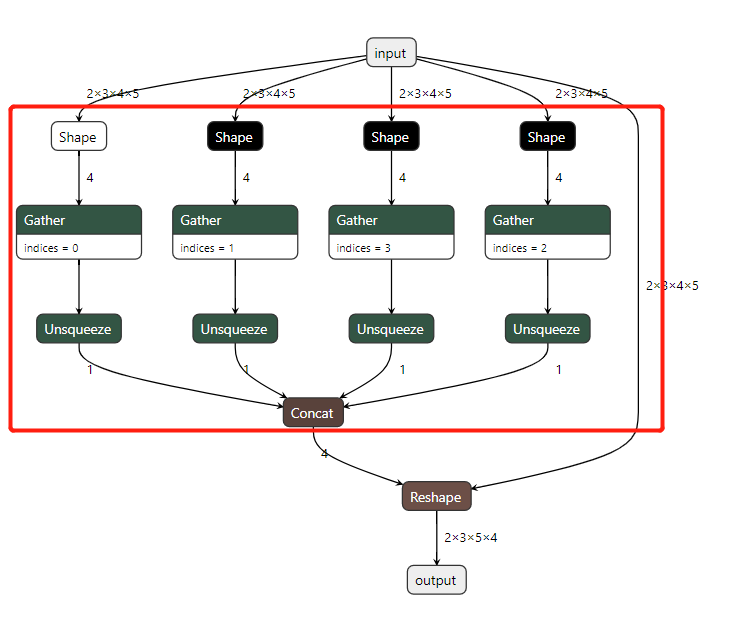def forward_for_node_outputs(model: onnx.ModelProto, nodes: List[onnx.NodeProto],
input_shapes: Optional[TensorShapes] = None) -> Dict[str, np.ndarray]:
if input_shapes is None:
input_shapes = {}
model = copy.deepcopy(model)
# nodes 是Graph中所有的静态OP
res = forward(model, input_shapes=input_shapes)
return res


def add_features_to_output(m: onnx.ModelProto, nodes: List[onnx.NodeProto]) -> None:
"""
Add features to output in pb, so that ONNX Runtime will output them.
:param m: the model that will be run in ONNX Runtime
:param nodes: nodes whose outputs will be added into the graph outputs
"""
# ONNX模型的graph扩展输出节点，获取所有静态OP的输出和原始输出节点的输出
for node in nodes:
for output in node.output:
m.graph.output.extend([onnx.ValueInfoProto(name=output)])


def clean_constant_nodes(const_nodes: List[onnx.NodeProto], res: Dict[str, np.ndarray]):
"""
It seems not needed since commit 6f2a72, but maybe it still prevents some unknown bug
:param const_nodes: const nodes detected by get_constant_nodes
:param res: The dict containing all tensors, got by forward_all
:return: The constant nodes which have an output in res
"""
return [node for node in const_nodes if node.output in res]def eliminate_const_nodes(model: onnx.ModelProto, const_nodes: List[onnx.NodeProto],
res: Dict[str, np.ndarray]) -> onnx.ModelProto:
"""
:model参数: 原始ONNX模型
:const_nodes参数: 使用get_constant_nodes获得的静态OP
:res参数: 包含所有输出Tensor的字典
:return: 简化后的模型. 所有冗余操作都已删除.
"""
for i, node in enumerate(model.graph.node):
if node in const_nodes:
for output in node.output:
new_node = copy.deepcopy(node)
new_node.name = "node_" + output
new_node.op_type = 'Constant'
new_attr = onnx.helper.make_attribute(
'value',
onnx.numpy_helper.from_array(res[output], name=output)
)
del new_node.input[:]
del new_node.attribute[:]
del new_node.output[:]
new_node.output.extend([output])
new_node.attribute.extend([new_attr])
insert_elem(model.graph.node, i + 1, new_node)
del model.graph.node[i]

return model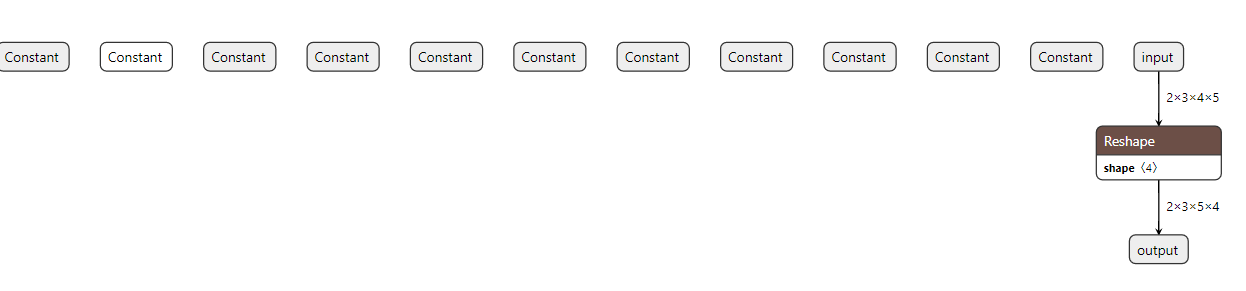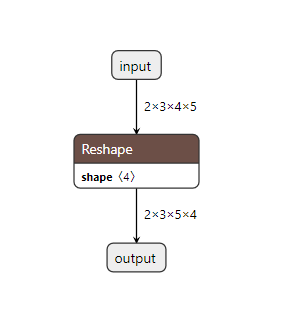# 0x7. 参考资料¶